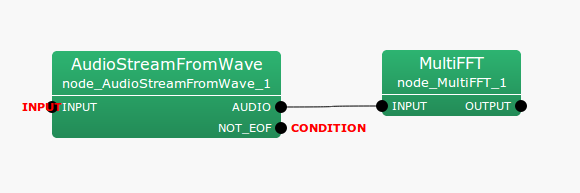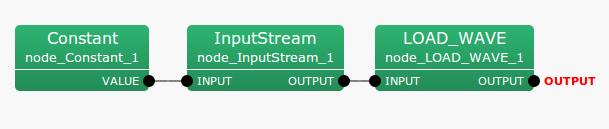## 6.1.2 AudioStreamFromWave

### 6.1.2.1 Outline of the node

This node reads speech waveform data from a WAVE file. The waveform data is read into a Matrix<float>  type: indexed, multichannel audio waveform data with rows as channels and columns as samples.

### 6.1.2.2 Necessary file

Audio files in RIFF WAVE format. There are no limits for the number of channels and sampling frequency. For quantization bit rates, 16-bit or 24-bit signed integers linear PCM format are assumed.

### 6.1.2.3 Usage

When to use

This node is used when wishing to use WAVE files as input to the HARK system

Typical connection

Figures 6.7 and 6.8 show an usage example of the AudioStreamFromWave node. Figure 6.7 shows an example of AudioStreamFromWave  converting Matrix<float>  type multichannel waveforms read from a file into frequency domain with the MultiFFT  node. To read a file with AudioStreamFromWave , designate a filename in Constant  node (Normal node FlowDesigner) and generate a file descriptor in the InputStream  node as shown in Figure 6.8. Further, connect the output of the InputStream  node to the iterator subnetwork(LOAD_WAVE in Figure 6.8), which contains networks of various nodes of HARK such as AudioStreamFromWave .Figure 6.7: Connection example of AudioStreamFromWave  1Figure 6.8: Connection example of AudioStreamFromWave  2

### 6.1.2.4 Input-output and property of node

Table 6.3: Parameter list of AudioStreamFromWave
 Parameter name Type Default value Unit Description LENGTH 512 [pt] Frame length as a fundamental unit for processing. ADVANCE 160 [pt] Frame shift length. USE_WAIT false Designate if processing is performed in real time

Input

INPUT

: Stream  type. Receive inputs from the InputStream  node in IO category of FlowDesigner standard node.

Output

AUDIO

: Matrix<float>  type. Indexed, multichannel audio waveform data with rows as channels and columns as samples. The number of columns is equal to the parameter LENGTH.

NOT_EOF

: bool  type. Indicate if the file can still be read. Used as an ending flag for loop processing of files. When reaching the end of file, its outputs false and outputs true in other cases.

Parameter

LENGTH

: int  type. The default value is 512. Designates the frame length, which is a base unit of processing, in terms of number of samples. The higher the value, the higher the frequency resolution, but the lower the temporal resolution. It is known that length corresponding to $20 \sim 40$ [ms] is appropriate for the analysis of audio waveforms. The default value of 32 [ms] corresponds to the sampling frequency 16,000 [Hz].

Rough indication of parameters: When the goal of processing is speech analysis (speech recognition), about $20 \sim 40$ [ms] would be appropriate for LENGTH and $1/3 \sim 1/2$ of LENGTH would be appropriate for ADVANCE. In the case that sampling frequency is 16000 [Hz], the default values of LENGTH and ADVANCE are 32 and 10 [ms], respectively.